# Homework Given February 20, 2007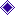Due February 27, 2007Chapter 2 exercises are on pages 87 through 105.

## Live Links to the ExercisesExercise 3.1Exercise 3.21Exercise 3.44Exercise 3.62Exercise 3.85

## Exercise 3.1

A 3 mA current source feeds square-law device with parameters A=1.5 mA/V2 and VTR=2 V.  Find the voltage vS across the square-law device.

This problem is in a block on Section 3.1, pages 107-110.  There is an equation on page 108, Equation (3.1), that expresses the v-i characteristic of the square-law device.  We are driving it with a current source, so we are forcing a current.  For nonzero current, the equation for the v-i curve is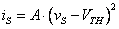Since the known is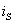and the unknown is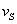, we solve for: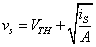For the parameters given in the problem statement,## Exercise 3.21

We have a 5 V source and a voltage divider, with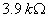and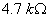, and a forward-biased diode across theresistor.  We see immediately that the voltage divider will produce more than the turn-on voltage of 0.7 V for the silicon diode given in Figure 3.18 on page 120, so the diode will conduct.  The simplest way to approach the problem is to find the Thévenin equivalent circuit for the source and voltage divider and use its v-i curve as a load line on Figure 3.18.  The Thévenin voltage is, the Thévenin resistance is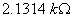, and the short circuit current is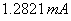.

With the Thévenin equivalent circuit, we run the load line on Figure 3.18 (actually, we use Excel’s Goal Seek utility with the parameters as given in the text below Figure 3.18) to find that the diode voltage is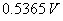and the diode current is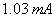.  Thus we have the solution for part (a).

Part (b) asks what the solution is if the source is changed to 10 V.  We find that the Thévenin voltage is now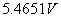, the Thévenin resistance remains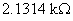and the short circuit current is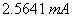.  A load line (or Excel Goal Seek) gives us a new diode voltage of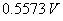and a diode current of.

Part (c) asks what happens if the diode current is reversed, with the source set at 5 V.  The diode is back-biased, and the diode current is the saturation current of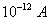.  This current is so small that its loading on the Thévenin circuit is negligible, so the diode voltage is the Thévenin open circuit voltage,.

## Exercise 3.44

This problem requires us to find the diode voltage as a function of current.  Thus we invert the diode equation as given in the problem statement and in Equation 3.20 on page 120,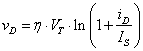.

Given this equation and the parameters in the problem statement,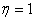,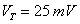and, we can use this equation to construct the table

Table 1.  Values of Diode Voltage for Problem 3.44

 iD vD -2E-12 #NUM! -9.9E-13 -0.11513 -9E-13 -0.05756 -5E-13 -0.01733 0 0 0.000001 0.345388 0.001 0.518082 0.01 0.575646 1 0.690776

Note that there is a numerical error when we force a reverse current in excess of the saturation current.  This is because the diode must break down to accept this current, and we are in the avalanche breakdown region that is not treated by the equation we are using.

## Exercise 3.62

We are asked to use the v-i curves given in Figure 3.47 page 147.  In the three cases we are asked to treat, we are driving the Zener diode with a voltage source in series with a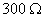resistor.

In part (a), the Zener diode is reverse-biased, the source is 9 V, so the short circuit current is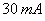.  We are clearly in the reverse breakdown region, with a diode voltage of about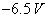.

In part (b), the source is reduced to 6 V, giving us a short circuit current of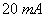.  The load line tells us that we are operating the Zener diode just barely over its breakdown voltage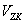so that we are technically in the reverse breakdown region, although common sense tells us that we are on a transition part of the Zener v-i curve between the reverse bias and reverse breakdown regions.

In part (c), we add a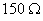resistor in parallel with the Zener diode, and we have a 9 V source.  Our new Thévenin equivalent voltage is 3 V, so the Zener cannot be brought into the reverse breakdown region.  The current through the Zener is its saturation current of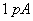and the diode voltage is 3 V.

## Exercise 3.85

Here we have a source of 10 V driving a circuit through a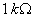resistor.  Both diodes in Figure P3.85 on page 164 are forward biased and our source voltage is far greater than the forward voltage of either diode, , so we know that at least one of the diodes is conducting.  This block of problems is for Section 3.3.6, Schottky Diode, on pages 129 and 130.  In that section, only the constant voltage drop diode models are used, so we will use the constant voltage drop diode model here.  The first diode D1 is given as having a forward voltage of 0.7 V and the Schottky diode D2 is given as having a forward voltage of 03 V.

Looking only at the first diode, D1, we see that we have 9.3 V across the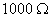resistor R1, so that the current through R1 is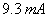, and that D1 is conducting.  This represents a voltage source at D1 of 0.7 V, which is the equivalent circuit presented to the remainder of the circuit.

The voltage of 0.7 V is presented to R2 which has a value ofand the Schottky diode D2.  The voltage across R2 is the difference in the forward voltages of the two diodes, 0.4 V, so the current through this circuit is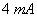by Ohm’s law.

Since the portion of the circuit with D2 drainsand the current through R1 is, the KCL tells us that the current through D1 is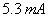.